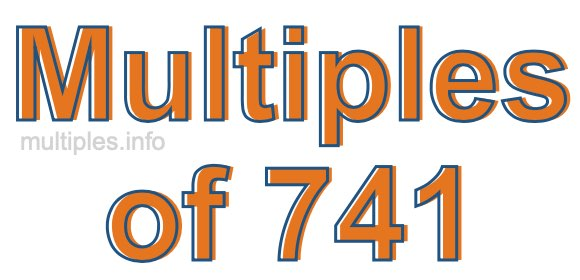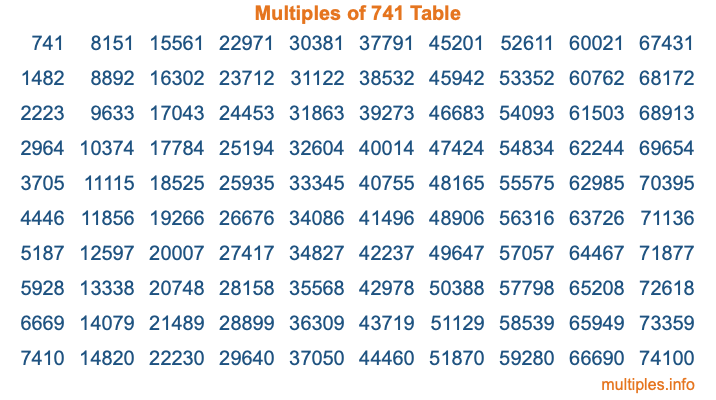Multiples of 741Welcome to the Multiples of 741 page. Here we will first teach you everything you will ever need to know about the multiples of 741, and then give you a study guide summary of everything we taught you to make sure you remember it all. Use this page to look up facts and learn information about the multiples of 741. This page will make you a multiples of seven hundred forty-one expert!

Definition of Multiples of 741
Multiples of 741 are all the numbers that when divided by 741 equal an integer. Each of the multiples of 741 are called a multiple. A multiple of 741 is created by multiplying 741 by an integer.

Therefore, to create a list of multiples of 741, you start with 1 multiplied by 741, then 2 multiplied by 741, then 3 multiplied by 741, and so on for as long as you want. Thus, the list of the first five multiples of 741 is 741, 1482, 2223, 2964, and 3705. To see a larger list of multiples of 741, see the printable image of Multiples of 741 further down on this page. We also have a category where you can choose any nth multiple of 741.

Multiples of 741 Checker
The Multiples of 741 Checker below checks to see if any number of your choice is a multiple of 741. In other words, it checks to see if there is any number (integer) that when multiplied by 741 will equal your number. To do that, we divide your number by 741. If the the quotient is an integer, then your number is a multiple of 741.

Is  a multiple of 741?

Least Common Multiple of 741 and ...
A Least Common Multiple (LCM) is the lowest multiple that two or more numbers have in common. This is also called the smallest common multiple or lowest common multiple and is useful to know when you are adding our subtracting fractions. Enter one or more numbers below (741 is already entered) to find the LCM.

Check out our LCM Calculator if you need more details about the Least Common Multiple or if you need the LCM for different numbers for adding and subtraction fractions.

nth Multiple of 741
As we stated above, 741 is the first multiple of 741, 1482 is the second multiple of 741, 2223 is the third multiple of 741, and so on. Enter a number below to find the nth multiple of 741.

th multiple of 741

Multiples of 741 vs Factors of 741
741 is a multiple of 741 and a factor of 741, but that is where the similarities end. All postive multiples of 741 are 741 or greater than 741. All positive factors of 741 are 741 or less than 741.

Below is the beginning list of multiples of 741 and the factors of 741 so you can compare:

Multiples of 741: 741, 1482, 2223, 2964, 3705, etc.

Factors of 741: 1, 3, 13, 19, 39, 57, 247, 741

As you can see, the multiples of 741 are all the numbers that you can divide by 741 to get a whole number. The factors of 741, on the other hand, are all the whole numbers that you can multiply by another whole number to get 741.

It's also interesting to note that if a number (x) is a factor of 741, then 741 will also be a multiple of that number (x).

Multiples of 741 vs Divisors of 741
The divisors of 741 are all the integers that 741 can be divided by evenly. Below is a list of the divisors of 741.

Divisors of 741: 1, 3, 13, 19, 39, 57, 247, 741

The interesting thing to note here is that if you take any multiple of 741 and divide it by a divisor of 741, you will see that the quotient is an integer.

Multiples of 741 Table
Below is an image of the first 100 multiples of 741 in a table. The table is in chronological order, column by column. The first column has the first ten multiples of 741, the second column has the next ten multiples of 741, and so on.The Multiples of 741 Table is also referred to as the 741 Times Table or Times Table of 741. You are welcome to print out our table for your studies.

Negative Multiples of 741
Although not often discussed or needed in math, it is worth mentioning that you can make a list of negative multiples of 741 by multiplying 741 by -1, then by -2, then by -3, and so on, to get the following list of negative multiples of 741:

-741, -1482, -2223, -2964, -3705, etc.

Multiples of 741 Summary
Below is a summary of important Multiples of 741 facts that we have discussed on this page. To retain the knowledge on this page, we recommend that you read through the summary and explain to yourself or a study partner why they hold true.

There are an infinite number of multiples of 741.

A multiple of 741 divided by 741 will equal a whole number.

741 divided by a factor of 741 equals a divisor of 741.

The nth multiple of 741 is n times 741.

The largest factor of 741 is equal to the first positive multiple of 741.

741 is a multiple of every factor of 741.

741 is a multiple of 741.

A multiple of 741 divided by a divisor of 741 equals an integer.

741 divided by a divisor of 741 equals a factor of 741.

Any integer times 741 will equal a multiple of 741.

Multiples of a Number
Here you can get the multiples of another number, all with the same attention to detail as we did for multiples of 741 on this page.

Multiples of
Multiples of 742
Did you find our page about multiples of seven hundred forty-one educational? Do you want more knowledge? Check out the multiples of the next number on our list!